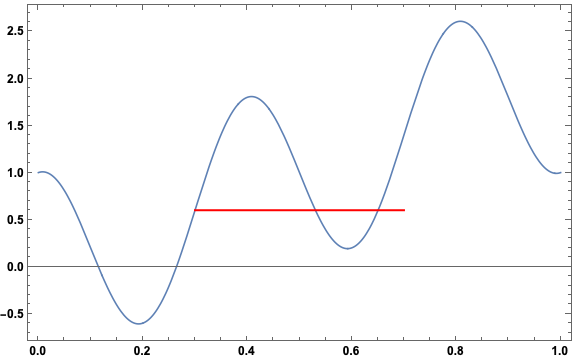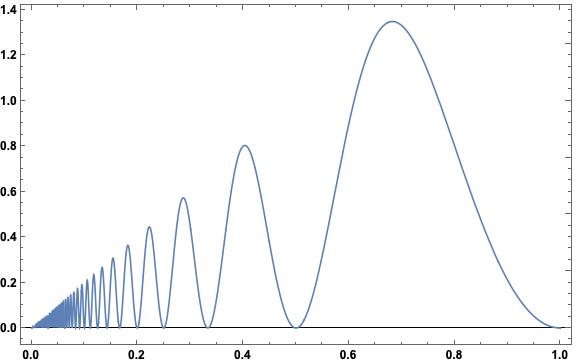# A Quasiperiodic Counterexample

## The problem

Consider a function $$f: [0, 1] \to \mathbb{R}$$ that is continuous with $$f(0) = f(1)$$. It is possible to prove that for each $$n \in \mathbb{N}$$, there exist $$x_n, y_n \in [0, 1]$$ such that $$|x_n - y_n| = 1/n$$ and $$f(x_n) = f(y_n)$$. This means the set of points at which the function is not one-to-one is at least countably infinite. Providing the proof is part of Exercise 4.5.6 in Abbott’s Understanding Analysis (2nd edition).

I’m not going to discuss the proof here, but I am interested in the final part of the exercise, which asks for a specific counterexample. Quoting Abbott:

If $$h \in (0, 1)$$ is not of the form $$1/n$$, there does not necessarily exist $$|x - y| = h$$ satisfying $$f(x) = f(y)$$. Provide an example that illustrates this using $$h = 2/5$$.

I found this perhaps harder than it should have been. Restating the problem slightly, we are looking for a function $$f$$ such that $$f(x + 2/5) - f(x) \neq 0$$, for all $$x \in [0, 3/5]$$ (since $$3/5 = 1 - 2/5$$), while satisfying the requirement that $$f(0) = f(1)$$.

## A specific counterexample

After some trial and error, I hit on the idea of using a function that does have a period of $$2/5$$, and then modifying it somehow. Starting with $$\sin$$: $\sin\left(\frac{5}{2}2\pi x\right)$ has a period of $$2/5$$, since $\sin\left[\frac{5}{2}2\pi \left(x + \frac{2}{5}\right)\right] = \sin\left(\frac{5}{2} 2\pi x + 2 \pi\right) = \sin\left(\frac{5}{2}2 \pi x\right).$

Now for the desired function $$f$$, try a function of the form $f(x) = \sin\left(\frac{5}{2}2\pi x\right) + a x .$ Requiring that $$f(0) = f(1)$$ means that $a = - \sin\left(\frac{5}{2}2\pi\right) = 0,$ so this functional form won’t work.

Instead of $$\sin$$, lets try $$\cos$$: $\cos\left(\frac{5}{2}2\pi x\right)$ has a period of $$2/5$$, and trying the same kind of functional form: $f(x) = \cos\left(\frac{5}{2}2\pi x\right) + a x$, we see that $$f(0) = 1$$ and $$f(1) = -1 + a$$, so $$a = 2$$ works. Calculating the difference $f(x + \frac{2}{5}) - f(x) = 2 \left(x + \frac{2}{5}\right) - 2x = \frac{4}{5},$ so this is the desired counterexample! Here is a plot, along with a horizontal red line of length $$2/5$$ shown at one point along the function:Imagine sliding the red line around on the function (keeping it horizontal). The two ends of the line never simultaneously interesect the function! In fact, a little experimentation shows that the line is not optimal, in the sense that it could be a bit shorter or a bit longer and still not intersect the function at both ends. So our counterexample $$f(x)$$ is a counterexample for values of $$h$$ close to $$2/5$$ as well as for $$h = 2/5$$.

## Generalizing

The fact that the above function $$f(x)$$ is a counterexample for values of $$h$$ close to $$2/5$$ shows that there is nothing special about $$2/5$$. Specifically there is nothing special about the fact that it is rational. So let’s try to find a function for arbitrary values of $$h \in (0, 1)$$.

First, note that we can take care of many values of $$h$$ at once by using the simple function $f(x) = \sin(2\pi x).$ If $$h > 1/2$$ and $$x < 1/2$$ then $$f(x + h) = -f(x)$$, so $$f(x + h) - f(x) = -2 f(x) < 0$$. We don’t have to consider larger values of $$x$$ since the property $$f(x + h) \neq f(x)$$ only needs to hold for $$x \in [0, 1 - h]$$. This shows that there does not necessarily exist $$h \in (1/2, 1)$$.

For arbitrary $$h \in (0, 1/2)$$, let’s try a function of the same form as the specific counterexample above: $f(x) = \cos\left(\frac{2\pi x}{h}\right) + a x.$ Then $$f(0) = 1$$ and $$f(1) = \cos\left(\frac{1}{h}2\pi\right) + a$$, so the desired form is $\boxed{ f(x) = \cos\left(\frac{2\pi x}{h}\right) + \left(1 - \cos\left(\frac{2\pi}{h}\right)\right)x .}$

Calculating the difference: $f(x + h) - f(x) = h \left(1 - \cos\left(\frac{2\pi}{h}\right)\right) = 2 h \sin^2\left(\frac{\pi}{h}\right),$ using a half-angle formula.

The difference is greater than zero for all values of $$h$$ except for $$h$$ such that $$\sin(\pi/h) = 0$$, which are exactly the values $$1/n, n \in \mathbb{N}$$ for which there is always at least one place where the function is not one-to-one!

Here is a plot of the difference $$f(x + h) - f(x) = 2 h \sin^2\left(\frac{\pi}{h}\right)$$ as a function of $$h$$:It only intersects zero at the countably infinite set of points $$1/n, n \in \mathbb{N}$$.

## Summary

Any continuous function $$f:[0, 1] \to \mathbb{R}$$ satisfying $$f(0) = f(1)$$ must fail to be one-to-one for at least one pair of points in $$[0, 1]$$ separated by $$h = 1/n$$, and this is true for all $$n \in \mathbb{N}$$. Furthermore, by the counterexample given above, there are no other “separations” $$h \in (0, 1)$$ for which non-injectivity is guaranteed!

Is there another general functional form that provides a counterexample for arbitrary values of $$h \neq 1/n$$? Let me know if you find one!##### Landon Lehman
###### Data Scientist

My research interests include data science, statistics, physics, and applied math.